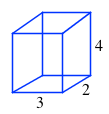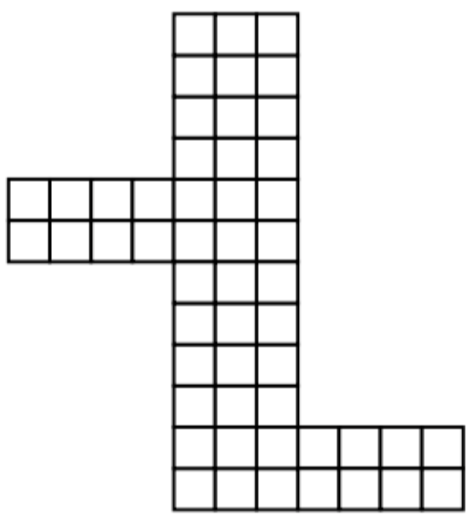### Home > GC > Chapter 12 > Lesson 12.2.4 > Problem12-93

12-93.

Examine the net at right.

1. Describe the solid that is formed by this net. What are its dimensions?

It is a prism with dimensions $2 \times 3 \times 4$ units.2. Find the surface area and volume of the solid formed by this net.

To find the surface area, count the number of boxes in the net figure.
To find the volume multiply the dimensions you found in part (a).

3. If all the dimensions of this solid are multiplied by $3$, what is the SA of the resulting solid? What is the volume?

$\text{Surface area}=\left(52\right)\left(3\right)^2$
$\text{Volume}=\left(24\right)\left(3\right)^3$